# Exponential distribution

Jump to: navigation, search

2010 Mathematics Subject Classification: Primary: 60E99 [MSN][ZBL]

A continuous distribution of a random variabledefined by the density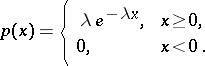(1)

The density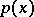is dependent on the positive scale parameter. The formula for the moments is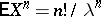, and, in particular, the expectation equals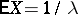and the variance equals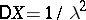; the characteristic function is.

The exponential distribution belongs to the family of gamma-distributions (cf. Gamma-distribution) which are defined by the densities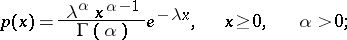the-fold convolution of the density (1) is equal to the gamma-density with the same parameterand with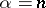.

The exponential distribution is the unique distribution having the property of no after-effect: For any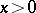,one has(2)

where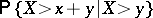is the conditional probability of the event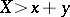subject to the condition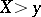. Property (2) is also called the lack-of-memory property.

In a homogeneous Poisson process, the distances between successive events have an exponential distribution. Conversely, a renewal process with exponential lifetime (1) is a Poisson process. An exponential distribution often arises as a limit process on the superposition or extension of renewal processes, as well as in high-level intersection problems in various random-path schemes, in critical branching processes, etc.

The above features explain why the exponential distribution is widely used in calculating various systems in queueing theory and reliability theory. One assumes that the lifetimes of the devices are independent random variables with exponential distributions, and then the property (2) enables one to examine a queueing system by means of finite or denumerable Markov chains with continuous time. Similarly, one uses Markov chains in reliability theory, where the fault-free operating times of the individual devices can often be taken as independent and as having exponential distributions.